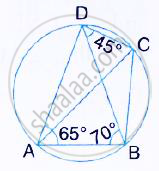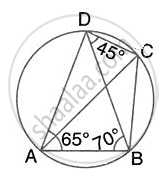Share

# In the Given Figure, ∠Bad = 65°, ∠Abd = 70° and ∠Bdc = 45°. Find: ∠Bcd Hence, Show that Ac is a Diameter - Mathematics

Course

#### Question

In the given figure, ∠BAD = 65°, ∠ABD = 70° and ∠BDC = 45°. Find: ∠BCD
Hence, show that AC is a diameter#### Solution∠BCD = 180° - ∠BAD = 180° - 65° = 115°

(pair of opposite angles in a cyclic quadrilateral are supplementary)

Is there an error in this question or solution?